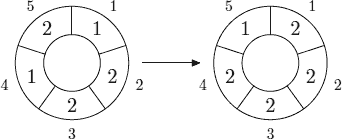# 「POJ3150」Cellular Automaton

2014年3月4日3,5570

Description

cellular automaton is a collection of cells on a grid of specified shape that evolves through a number of discrete time steps according to a set of rules that describe the new state of a cell based on the states of neighboring cells. The order of the cellular automaton is the number of cells it contains. Cells of the automaton of order n are numbered from 1 to n.

The order of the cell is the number of different values it may contain. Usually, values of a cell of order m are considered to be integer numbers from 0 to m − 1.

One of the most fundamental properties of a cellular automaton is the type of grid on which it is computed. In this problem we examine the special kind of cellular automaton — circular cellular automaton of order n with cells of order m. We will denote such kind of cellular automaton as n,m-automaton.

A distance between cells i and j in n,m-automaton is defined as min(|i − j|, n − |i − j|). A d-environment of a cell is the set of cells at a distance not greater than d.

On each d-step values of all cells are simultaneously replaced by new values. The new value of cell i after d-step is computed as a sum of values of cells belonging to the d-enviroment of the cell i modulo m.

The following picture shows 1-step of the 5,3-automaton.The problem is to calculate the state of the n,m-automaton after k d-steps.

Input

The first line of the input file contains four integer numbers nmd, and k (1 ≤ n ≤ 500, 1 ≤ m ≤ 1 000 000, 0 ≤ d < n2 , 1 ≤ k ≤ 10 000 000). The second line contains n integer numbers from 0 to m − 1 — initial values of the automaton’s cells.

Output

Output the values of the n,m-automaton’s cells after k d-steps.

Sample Input

Sample Output

1 2 2 1 2

5 5 5 5 4

a0 a1 a2 a3 a4
->(a4+a0+a1) (a0+a1+a2) (a1+a2+a3) (a2+a3+a4) (a3+a4+a0)

b = 1 2 2 1 2
a =
1 1 0 0 1
1 1 1 0 0
0 1 1 1 0
0 0 1 1 1
1 0 0 1 1
b * a = 5 5 5 5 4Subscribe